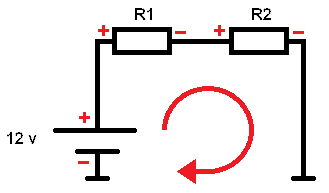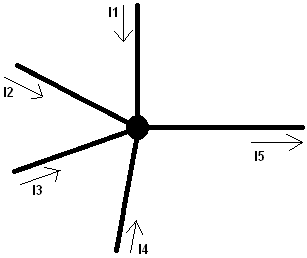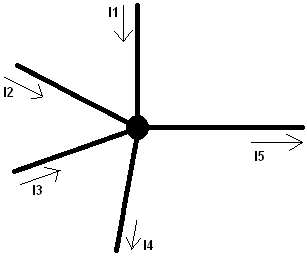# kirchoff

Subjects:

• Kirchhoff's voltage law
• Kirchhoff's current law

Kirchhoff's voltage law:
Kirchhoff's voltage law means that the sum of the electrical potential differences is equal to 0. Simply put, in a closed circuit, the input and output voltages are equal to 0. The following explanation is about the drawing below with 2 resistors:

In a closed circuit, the total current through the circuit can be calculated using the voltage and resistance values.
-12 + 5 x I + 10 x I = 0
(-12 volts + 5 ohms x the current + 10 ohms x the current = equal to 0)
Because this is a series circuit, the resistances can be added together; 5 + 10 = 15Ω. Then the current I can be calculated:

I= U / R
I = 12 / 15
I = 0,8A

The total current through the circuit is 0,8A. The current is the same from the battery as well as at R1 and R2. Now the voltage across the resistors has to be calculated. The current and resistance values ​​are known, so Ohm's law can now calculate the voltage:

UR1 and UR2 are the voltages (U) across the resistors R1 and R2):

UR1 = I x R
UR1 = 0,8 x 5
UR1 = 4v

UR2 = I x R
UR2 = 0,8 x 10
UR2 = 8v

Now Kirchhoff's law can be applied;
-Ubatt + UR1 + UR2 = 0
-12v + 4 + 8 = 0U = 12v
R1 = 5
R2 = 10
I = unknown

This proves that the Kirchhoff voltage equation is correct, because if you start at the battery at the bottom left of the diagram, you start at the – of the battery. That's why you start with -12. If you continue reading (clockwise) the diagram, you will first arrive at the + of R1 and then the + of R2. Hence – the voltage of the battery (input at the voltage source), is equal to (plus) the sum of all (outgoing) consumers. In this case the resistors. This can be a means of control in complex schemes where, for example, replacement resistances have been calculated. By applying Kirchhoff's voltage law, it is possible to check whether the calculated data is correct.

Kirchhoff's current law:
Kirchhoff's current law states that all currents in a node are equal to 0. All currents entering the node must also exit.

I1 + I2 + I3 + I4 = I5 (all currents exit the node via I5)With multiple flows exiting the node, the schema and formula become as follows:

I1 + I2 + I3 = I4 + I5 (the currents I1, I2 and I3 are distributed over I4 and I5).error: Alert: Content is protected !!